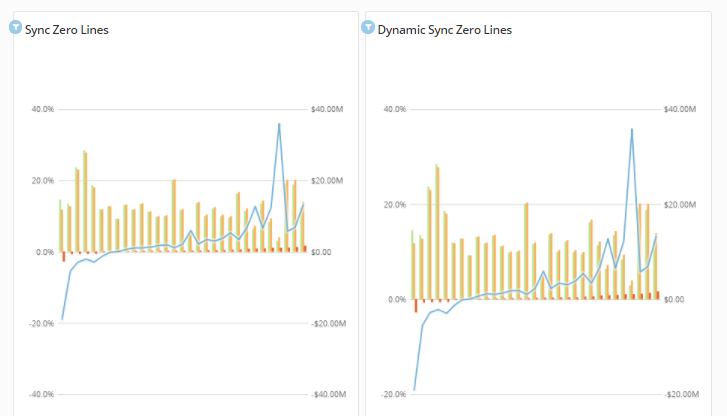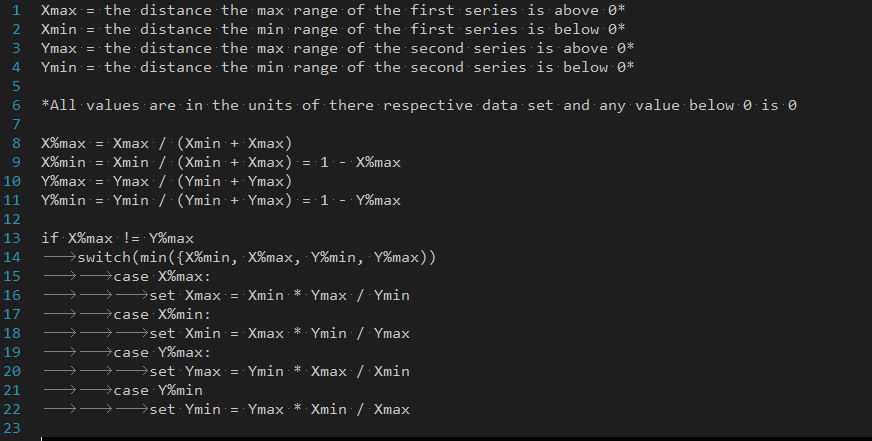# Sync Zero Lines By Match Ratio Above and Below 0 for both Ranges

Currently the "Sync Zero Lines" option is centering both zeros on each axis which leads to a lot of wasted space on the graph if either both sets have ranges that are mostly above zero or both sets have ranges that are mostly below zero.

Ideally it should sync the zero lines by moving the range value that is closest to 0 relative to the length of it's axis outwards until the ratios are equal.

In practice this would look similar to this...(The first fraph shows how this is currently handled)

Here is some psuedo code for how this would work...Tagged:
1